﻿ 过套管电阻率测井仪机械系统优化设计 Optimum Design of Mechanical System for Casing Resistivity Logging Tool

Mechanical Engineering and Technology
Vol. 08  No. 02 ( 2019 ), Article ID: 29580 , 9 pages
10.12677/MET.2019.82010

Optimum Design of Mechanical System for Casing Resistivity Logging Tool

Hua Huang, Xiaolei Li, Fei Xu

Research Institute of Petroleum Engineering Technology, Zhongyuan Oilfield Branch, Puyang HenanReceived: Mar. 8th, 2019; accepted: Mar. 27th, 2019; published: Apr. 3rd, 2019ABSTRACT

In order to solve the previous casing formation resistivity logging instrument of logging limitation, through the study of the resistivity logging principle and technology characteristics, combining with the logging instrument design experience of domestic and overseas, we research and design a new generation instrument. In the process of the detailed design and analysis of the pushing assembly’s main structure, an electrode assembly is designed, which has the advantages of easy installation, disassembly, replacement and insulation, and has the characteristic that electrode can be withdrawn in the fault state. At the same time, the mechanics and optimization design models of pushing device are established, with the maximum logging diameter and minimum demand driving force, and the pushing device is optimized effectively based on complex method. In addition, hydraulic transmission system is designed on the premise that the pushing assembly’s working condition is analyzed in detail, which has the advantages of simple structure, complete function, pressure balance, position indicator and double safety overflow. And it can be simulated and analyzed with AMESim. Finally, the main performance parameters of the prototype are practically tested, including the force loaded by measuring electrode on casing, insulation among measuring electrodes, closing and opening consuming time of pushing device and temperature and pressure resistant performance of pushing assembly. As a result of the test, pushing assembly’s defect is discovered and design is improved. At the same time, the rationality of design and analysis of pushing assembly are fully validated by the test.

Keywords:Cased Hole Resistivity Logging, Four-Link Device, Pushing Assembly, Optimization DesignCopyright © 2019 by author(s) and Hans Publishers Inc.1. 引言

ECOS液压缸式推靠装置，通过液压力推挤活塞式的管内壁接触，并利用液压力维持电极探针对套管内壁的作用，最后通过泄压令电极探针收回，从而与套管内壁脱离。CHFR四连杆式推靠装置，采用板弹簧的形变回复力作为支撑电极探针与套管内壁接触的作用力，并通过作用在四连杆推靠臂施力端上的作用力，令四连杆推靠装置收回，从而促使电极探针与套管内壁脱离。相比较而言，CHFR的板弹簧回复力作用下的四连杆式推靠装置设计方案相比ECOS液压力作用下的液压缸式推靠装置更显“柔性”，使推靠装置更能适应不同的井况且不受到温度和液压油泄漏的影响。

2. 过套管电阻率测井原理

$\begin{array}{c}{R}_{d}=K\left(\frac{{\Delta }^{2}U\left({I}_{{А}_{1}}\right)-\Delta {U}_{{М}_{2}{М}_{1}}\left({I}_{{А}_{1}}\right)}{{I}_{{А}_{1}}}+\frac{{\Delta }^{2}U\left({I}_{{А}_{2}}\right)+\Delta {U}_{{М}_{2}{М}_{1}}\left({I}_{{А}_{2}}\right)}{{I}_{{А}_{2}}}\right)\\ \text{\hspace{0.17em}}\text{\hspace{0.17em}}×\left(\frac{{U}_{N}\left({I}_{{А}_{1}}\right)\cdot \Delta {U}_{{М}_{2}{М}_{1}}\left({I}_{2}\right)-{U}_{N}\left({I}_{{А}_{2}}\right)\cdot \Delta {U}_{{М}_{2}{М}_{1}}\left({I}_{1}\right)}{-\Delta {U}_{{M}_{2}{M}_{1}}\left({I}_{{A}_{1}}\right)\cdot {\Delta }^{2}U\left({I}_{{A}_{2}}\right)+\Delta {U}_{{M}_{2}{M}_{1}}\left({I}_{{A}_{2}}\right)\cdot {\Delta }^{2}U\left({I}_{{A}_{1}}\right)}\right)\end{array}$ (1)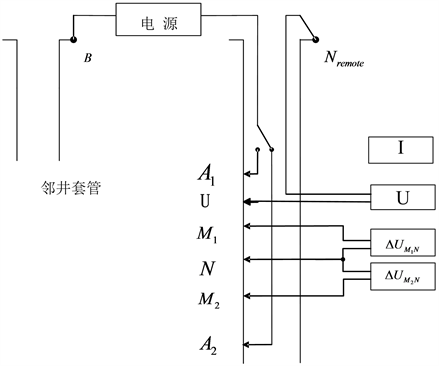Figure 1. Principle for through casing resistivity logging

3. 系统方案总体设计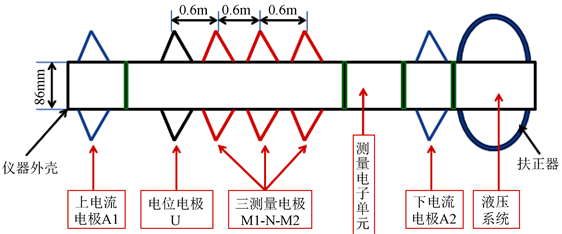Figure 2. Layout of push assembly for through-casing tool

4. 推靠总成优化参数设计与分析

4.1. 问题描述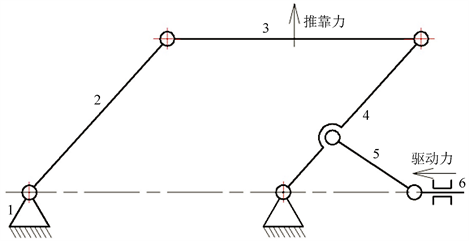1、机身；2、从动推靠臂；3、极板；4、主动推靠臂；5、连杆；6、驱动杆

Figure 3. Parallelogram pushing device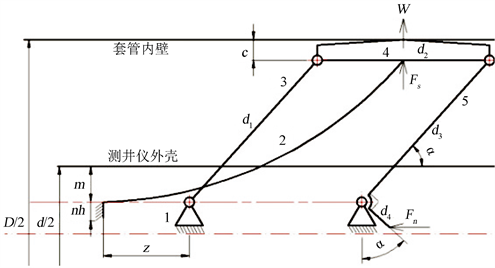Figure 4. Structure diagram of pushing device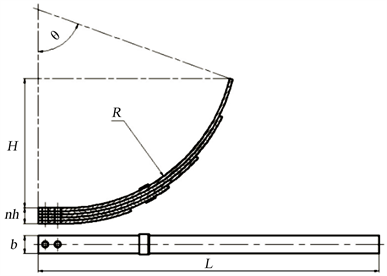Figure 5. Leaf spring of pushing device

4.2. 优化设计模型建立

4.2.1. 选取设计变量

$X=\left[{x}_{1},{x}_{2},{x}_{3},{x}_{4}\right]=\left[h,L,{d}_{3},{d}_{4}\right]$ (2)

4.2.2. 优化设计目标函数

1) 当推靠装置处于最大外张状态时，施加在推靠装置上的驱动力 ${F}_{\text{n}0}=0$ ，板弹簧的形变回复力 ${F}_{\text{s}0}$ ，则全部作用在极板上(推靠臂与测井仪中轴线的夹角表示为 ${\alpha }_{0}$ )，使其满足：

${F}_{\text{s0}}=W={f}_{0}k$ (3)

${f}_{0}=H+\frac{d}{2}-m-\frac{D}{2}+c$ (4)

${f}_{0}$ ——最大外张状态板弹簧的挠度，单位：mm。

2) 当推靠装置处于完全收拢状态时，板弹簧的最大形变回复力 ${F}_{\text{smax}}$ 和能将推靠装置完全收回的最小驱动力 ${F}_{\text{nmin}}$ ，满足(推靠臂与测井仪中轴线的夹角表示为 ${\alpha }_{\mathrm{min}}$ )：

${F}_{\text{smax}}={f}_{\mathrm{max}}k$ (5)

${f}_{\mathrm{max}}=H-\left(m-c\right)$ (6)

${f}_{\mathrm{max}}$ ——完全收拢状态板弹簧的挠度，单位：mm。

3) 最大测井直径D的函数：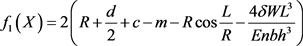(7)

${f}_{2}\left(X\right)=\frac{Enb{d}_{3}{h}^{3}}{4\delta {d}_{4}{L}^{3}}\left(R+c-m-R\mathrm{cos}\frac{L}{R}\right)$ (8)

$\mathrm{min}f\left(X\right)=\frac{{f}_{2}\left(X\right)}{{f}_{1}\left(X\right)}$ (9)

4.2.3. 确定约束函数

1) 静强度条件

${\sigma }_{\mathrm{max}}=\frac{3Eh{f}_{\mathrm{max}}}{2\delta {L}^{2}}\le \left[{\sigma }_{p}\right]$ (10)

${\sigma }_{\mathrm{max}}$ ——板弹簧的最大弯曲应力，MPa。

$\left[{\sigma }_{p}\right]$ ——板弹簧的许用弯曲应力，MPa。

2) 位置、尺寸约束

${H}_{0}={R}_{0}\left(1-\mathrm{cos}\frac{180L}{\text{π}{R}_{0}}\right)$ (11)

${H}_{0}=\frac{d}{2}-m-\frac{D}{2}+c$ (12)

${d}_{3}\mathrm{sin}{\alpha }_{0}+c=\frac{D}{2}-\left(\frac{d}{2}-m\right)$ (13)

$\sqrt{{R}_{0}^{2}-{\left({R}_{0}-{H}_{0}\right)}^{2}}=z+\frac{{d}_{2}}{2}+{d}_{3}\mathrm{cos}{\alpha }_{0}$ (14)

${H}_{0}$ ——最大外张状态板弹簧弧高，mm。

${R}_{0}$ ——最大外张状态板弹簧主片半径，mm。

${H}_{\mathrm{min}}={R}_{\mathrm{max}}\left(1-\mathrm{cos}\frac{180L}{\text{π}{R}_{\mathrm{max}}}\right)$ (15)

${H}_{\mathrm{min}}=m-c$ (16)

 (17)

$\sqrt{{R}_{\mathrm{max}}^{2}-{\left({R}_{\mathrm{max}}-{H}_{\mathrm{min}}\right)}^{2}}>z+{d}_{3}\mathrm{cos}{\alpha }_{\mathrm{min}}$ (18)

$2{d}_{3}\mathrm{cos}{\alpha }_{\mathrm{min}} (19)

${H}_{\mathrm{min}}$ ——完全收拢状态板弹簧弧高，mm。

${R}_{\mathrm{max}}$ ——完全收拢状态板弹簧主片半径，mm。

$l$ ——推靠总成的电极距，mm。本为取 $l=1200\text{\hspace{0.17em}}\text{mm}$

$\frac{b\sqrt{3}}{6}+nh+m<\frac{d}{2}$ (20)

$m+{d}_{4}<\frac{d}{2}$ (21)

4.3. 设计及优化结果分析Table 1. Optimization for structural parameters of push device

1386.7 N，比原设计减少了27.16%。由此说明，利用上述优化方法，成功地对推靠装置进行了优化，且优化效果明显。

5. 性能测试分析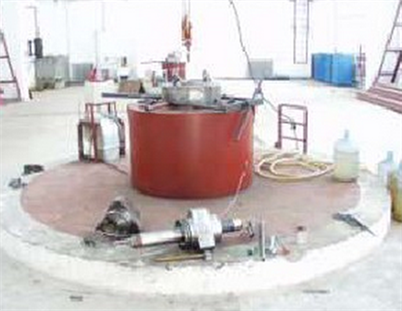Figure 6. High temperature and high pressure experimental device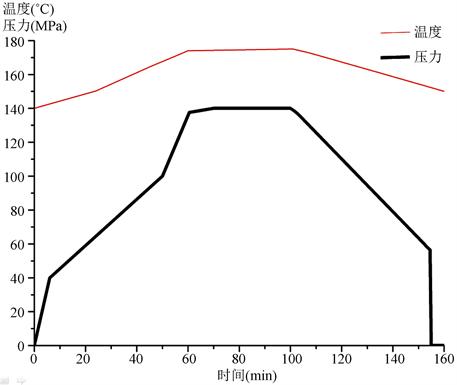Figure 7. Pressure record curve for through-casing tool

6. 结论

Optimum Design of Mechanical System for Casing Resistivity Logging Tool[J]. 机械工程与技术, 2019, 08(02): 71-79. https://doi.org/10.12677/MET.2019.82010

1. 1. 魏学良, 李俊杰, 曹卫东, 等. 过套管地层电阻率测井仪的研发设计[J]. 电子设计工程, 2017, 25(9): 87-91.

2. 2. 赵宏林, 陆应辉, 付国伟, 等. 过套管电阻率测井仪推靠机构优化设计[J]. 石油矿场机械, 2014, 43(9):37-41.

3. 3. 赵宏林, 梁华庆, 陆应辉, 等. 一种用于过套管电阻率测井仪中的可收回探针装置[P]. 中国专利, CN103821503A. 2014-08-13.

4. 4. 任尚华. 过套管地层电阻率测井仪的试制及现场试验[J]. 石油仪器, 2012, 26(4): 55-57.

5. 5. 张凤歧. 过套管电阻率测井在大庆油田剩余油监测上的应用分析[J]. 国外测井技术, 2013(6): 47-49.